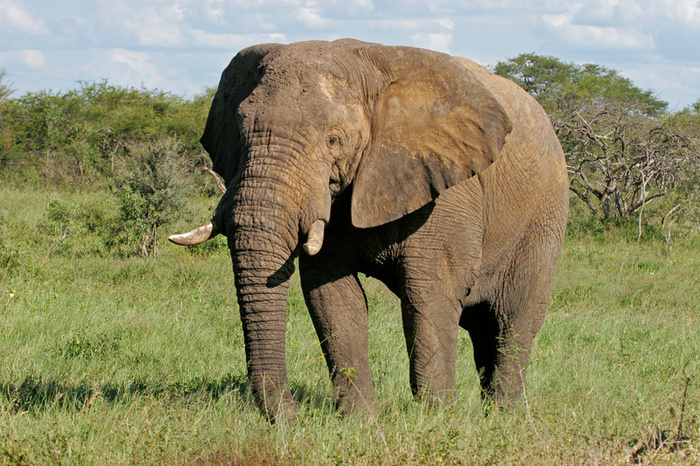# Larq Zoo Examples¶

This tutorial demonstrates how to load pretrained models from Larq Zoo. These models can be used for prediction, feature extraction, and fine-tuning.

import tensorflow as tf
import tensorflow_datasets as tfds
import numpy as np
import larq_zoo as lqz
from urllib.request import urlopen


In the following we will use a sample image from the ImageNet dataset:img_path = "https://raw.githubusercontent.com/larq/zoo/master/tests/fixtures/elephant.jpg"

with urlopen(img_path) as f:

x = tf.keras.preprocessing.image.img_to_array(img)
x = lqz.preprocess_input(x)
x = np.expand_dims(x, axis=0)


### Classify ImageNet classes with Bi-Real Net¶

We will first load the Bi-Real Net architecture with pretrained weights and predict the image class.

model = lqz.BiRealNet(weights="imagenet")
preds = model.predict(x)
lqz.decode_predictions(preds, top=5)

[('n02504458', 'African_elephant', 0.7529364),
('n01871265', 'tusker', 0.22607337),
('n02504013', 'Indian_elephant', 0.017037684),
('n02410509', 'bison', 0.0016636014),
('n02412080', 'ram', 0.0014974006)]


### Extract features with Bi-Real Net¶

Larq Zoo models can also be used to extract features that can be used as input to a second model.

tf.keras.backend.clear_session()
model = lqz.BiRealNet(weights="imagenet", include_top=False)
features = model.predict(x)
print("Feature shape:", features.shape)

Feature shape: (1, 7, 7, 512)


### Extract features from an arbitrary intermediate layer¶

Features can also be extracted from arbitrary intermediate layer with just a few lines of code.

avg_pool_layer = model.get_layer("average_pooling2d_2")
avg_pool_model = tf.keras.models.Model(
inputs=model.input, outputs=avg_pool_layer.output)

avg_pool_features = avg_pool_model.predict(x)
print("average_pooling2d_2 feature shape:", avg_pool_features.shape)

average_pooling2d_2 feature shape: (1, 7, 7, 256)


### Build Bi-Real Net over a custom input Tensor¶

The model can also be used with an input Tensor that might also be the output a different Keras model or layer.

input_tensor = tf.keras.layers.Input(shape=(224, 224, 3))

model = lqz.BiRealNet(input_tensor=input_tensor, weights="imagenet")


### Evaluate Bi-Real Net with TensorFlow Datasets¶

To re-run the evaluation on the entire ImageNet validation dataset Tensorflow Datasets can be used.

Note that running this example will download the entire dataset and might take a very long time to complete.

def preprocess(data):
img = lqz.preprocess_input(data["image"])
label = tf.one_hot(data["label"], 1000)
return img, label

dataset = (
.map(preprocess, num_parallel_calls=tf.data.experimental.AUTOTUNE)
.batch(128)
.prefetch(1)
)

model = lqz.BiRealNet()
model.compile(
optimizer="sgd",
loss="categorical_crossentropy",
metrics=["categorical_accuracy", "top_k_categorical_accuracy"],
)

model.evaluate(dataset)